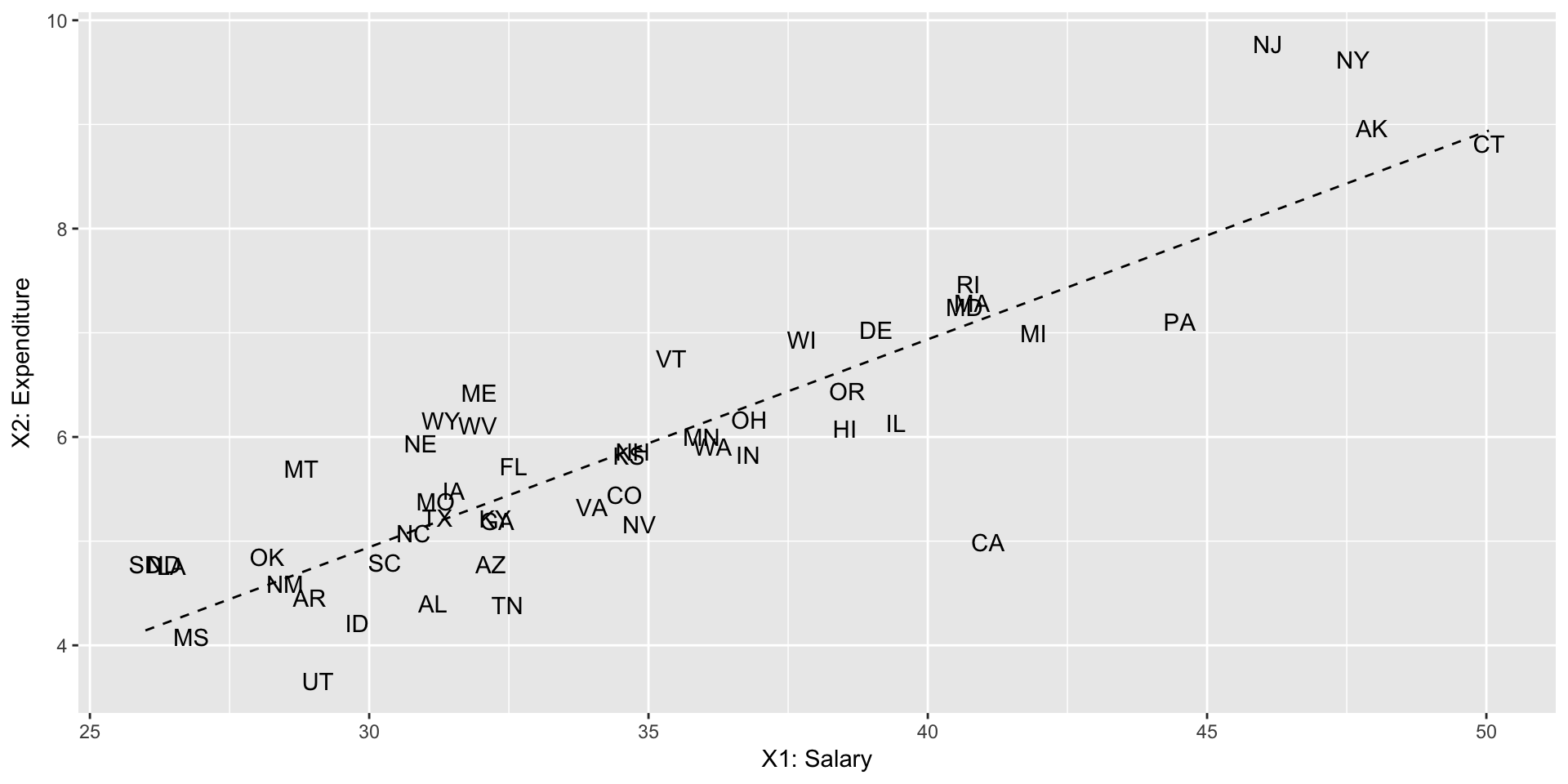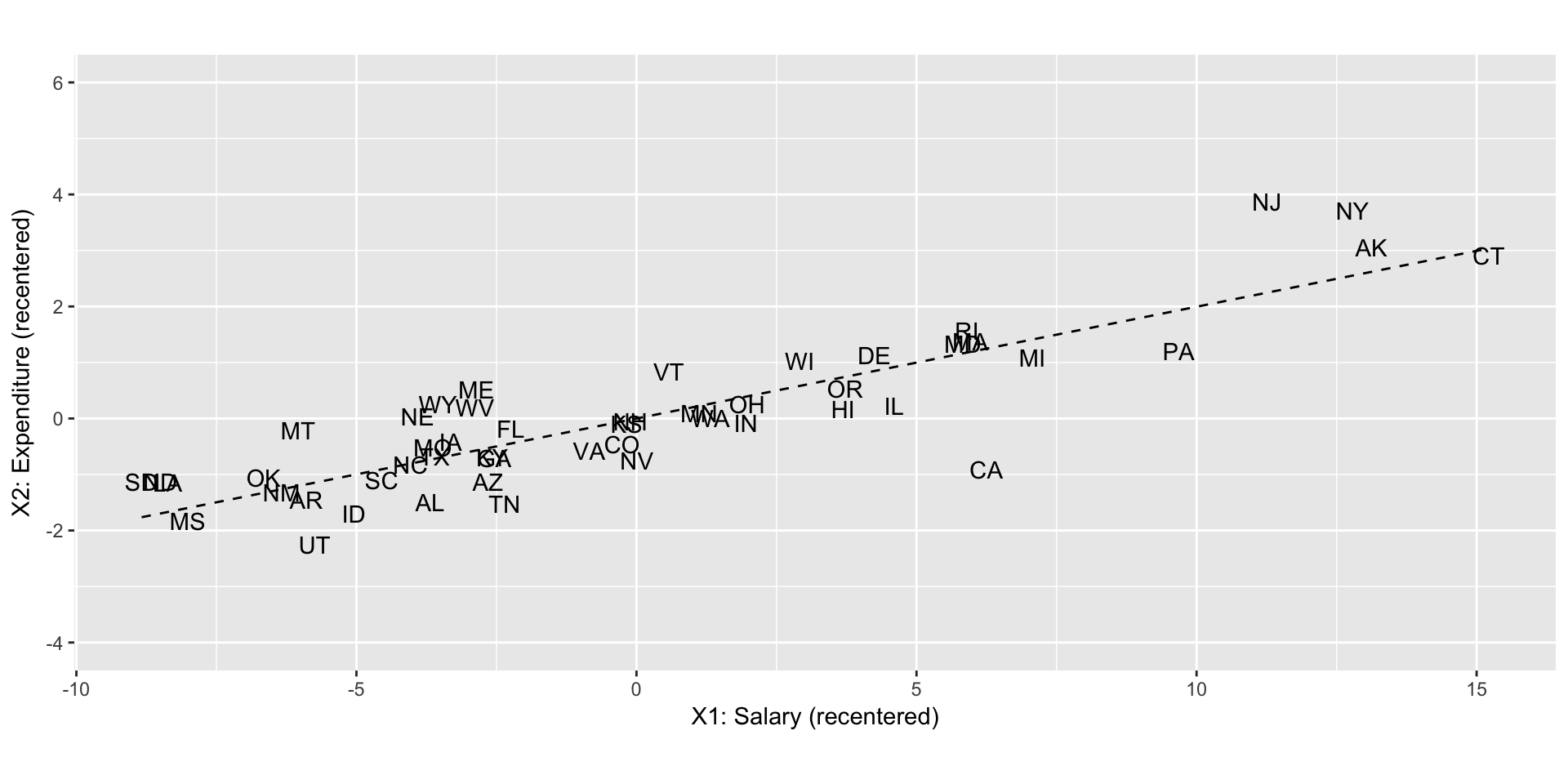## 1. Data

The data consists of SAT scores for the 50 states in 1994-1995:

library(tidyverse)
## Warning: package 'dplyr' was built under R version 3.4.2
SAT <- read_csv("http://people.reed.edu/~jones/141/sat.csv") %>%
select(State, salary, expend)
head(SAT)
State salary expend
Alabama 31.1 4.41
Arizona 32.2 4.78
Arkansas 28.9 4.46
California 41.1 4.99
X <- SAT %>%
select(salary, expend)

Let X be of dimension $$n \times p = 50 \times 2$$, where our observations $$\vec{X} = (X_1, X_2)$$ are

• $$X_1$$ salary: estimated average annual salary of teachers in public elementary and secondary schools (in $1000 of USD) • $$X_2$$ expend: expenditure per pupil in average daily attendance in public elementary and secondary schools (in$1000 of USD)

The two variables salary and expend are highly correlated ($$\rho=$$ 0.87). You can think of them as being redundant; once you know one variable, the other variable doesn’t provide you with all that more information. This is very apparent in the plot below (with the regression line being dashed):Note such collinear variables are a problem in a regression setting, because they “steal” each other’s effect, so it becomes difficult to isolate the effect of one vs the other. In other words, you have very unstable estimates of their $$\beta$$ coefficients/slopes.

## 2. Recentering Covariates

We first recenter both salary and expenditure to each have mean 0 by subtracting their sample means $$(\overline{x}_1, \overline{x}_2) = ( 34.829, 5.905)$$ respectively:

X_recenter <- X %>%
mutate(
salary = salary - mean(salary),
expend = expend - mean(expend)
)

We plot the two variables recentered at $$(0, 0)$$.We compute the sample/empirical $$2 \times 2$$ covariance matrix for both X and X_recenter (Note for students who have taken MATH310 Probability: they are identical since variances/covariances are invariant to linear transformations):

cov(X) %>% round(3)
##        salary expend
## salary  35.30   7.04
## expend   7.04   1.86
cov(X_recenter) %>% round(3)
##        salary expend
## salary  35.30   7.04
## expend   7.04   1.86

## 3. Principal Components

Using R’s built-in linear algebra functionlity, we

• Compute the first and second principal components. Linear algebra fact: they are the first and second eigenvectors $$\vec{\gamma}_1$$ & $$\vec{\gamma}_2$$ of the covariance matrix.
• Plot them in red and blue respectively.
eigen <- cov(X_recenter) %>% eigen()
eigen_vals <- eigen$values Gamma <- eigen$vectors